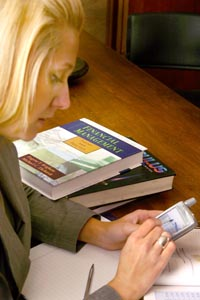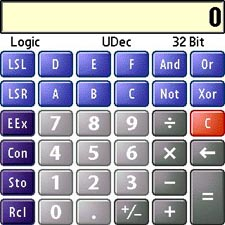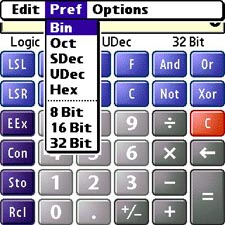treocentral.com >> Stories >> Guides
Hidden Treasures: The Treos' other calculators, Part 3
 Wed Aug 2, 2006 - 11:56 AM EDT - By Jay Gross

Overview### Our story so far

This is part 3 in the series on the PalmOS Treos� (650 and 700p) extra calculators. It documents the two remaining calculators, plus the four units converters. First we'll tackle Logic, then spend some quality time with Statistics, then we convert light years to hectares, pounds to pints, and miles to�well, you get the idea.

To review, check out Part 1, and Part 2. I made a couple of (gasp!) errors in those parts, so I�ll correct them in the Errata at the end of this article.

Now let�s explore the other Treo calculators. I�ve taken them up in the order in which they appear in the smart phones, and the next one on the list is ever so logical. Pun intended.

### LogicGeek alert! This exotic calculator performs math on hex numbers � or Binary, Octal, and other computer programmer favorites listed in the Prefs pulldown menu. It shifts right or left, and you can convert among hex, decimal, and any of the other choices. The actual �logic� functions are the four buttons at the far right, Boolean And, Or, Not, and Exclusive Or.

If you�re a programmer, you probably don�t need me to tell you how to use the Logic calculator, which has little practical use otherwise, but here goes:

```Example()
If
You <> programmer(computer)
OR You <> programmer(wannabe)
Then
Never_mind
Else
End If
```The Logic calculator puts plain and fancy arithmetic at the point of your Treo�s stylus. It can do math on numbers (floating point or integers), invoking a variety of conventions for displaying them. The numbers are the same, just expressed differently. The choices are listed in the Pref menu. Press the Menu button on the Treo and explore.

• Binary � If you need to know what this is, turn in your pocket protector at the front desk.
• Octal � This is base 8.
• SDec � Signed Decimal. Base 10, 2's complement format, most significant bit is the sign bit.
• Udec � Unsigned Decimal. Base 10, no sign bit.
• Hex � Base 16. That means 0 through F.

In the Logic calculator, the number base (radix) menu replaces the Float/Fixed/whatever menu that the other calculators have. At the bottom of that menu, you can change the integer size, 8, 16, or 32 bits. The calculator disables any number keys which don�t apply to the number base and integer size you�ve selected, and will refuse to accept numbers that are out of range of the integer size. So, in 8 bit format, you can enter 256, but not 257, and 32,268 is out of the question. The 32-bit integers go to 4,294,967,296, but after that, tough.

The calculator also converts among the number bases, applying limits as appropriate. If you need to look at Binary 0�s and 1�s for the number, change to the binary display. For example eleven (11) in Signed Decimal converts to 1011 in Binary. The Binary display is limited to the 33 rightmost digits � i.e., useful for numbers smaller than 2,147,483,647. You can convert larger numbers, but only 33 digits show � the �least significant bits� in programmer parlance.

In Hexadecimal � base 16 � the keypad comes up short. That�s what the ABCDEF keys are for. They don�t do anything in the other number bases.

### The functions

• LSL -- Logical Shift Left (shifts the value left by the number of bits you specify, and fills with zeros). Tap a number, tap LSL, tap the second number (the number of bits you want to shift by), and tap the big = button.
• LSR � Logical Shift Right. Visible in Bin, Octal, Unsigned Decimal, and Hex modes, LSR works the same as LSL, but shifts rightwards.
• ASR � Arithmetic Shift Right. Visible only in Signed Decimal mode, ASR indicates that the sign bit will be propagated rightwards.
• Plus/Minus � Changes the sign. In programmer-speak, this key performs 2's complement negation. For example, "1" changes to "FFFFFFFF" hex, the proper value for -1 in the standard 2's complement signed integer format. This is the same as doing "1[Not][ ]1[=]".
• AND -- Logical AND. Thank you Professor Bool.
• OR � Another Boolean
• NOT � Ditto.

The Store and Recall keys work the same as in the other calculators. In the Logic calculator, however, the values display in the current number base and integer size. The actual value in memory remains constant (unless you change it with Store), unaffected by displaying in other formats. Constants also display in the current number base, and can therefore be converted among them.

Logic isn�t for everyone, perhaps because it clearly dictates.

Statistics >>